The PRINCOMP Procedure
 Computational Resources

Let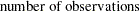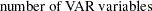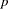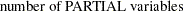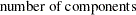• The minimum allocated memory required (in bytes) is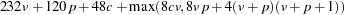• The time required to compute the correlation matrix is roughly proportional to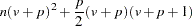• The time required to compute eigenvalues is roughly proportional to
.

• The time required to compute eigenvectors is roughly proportional to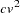.Previous Page | Next Page | Top of Page